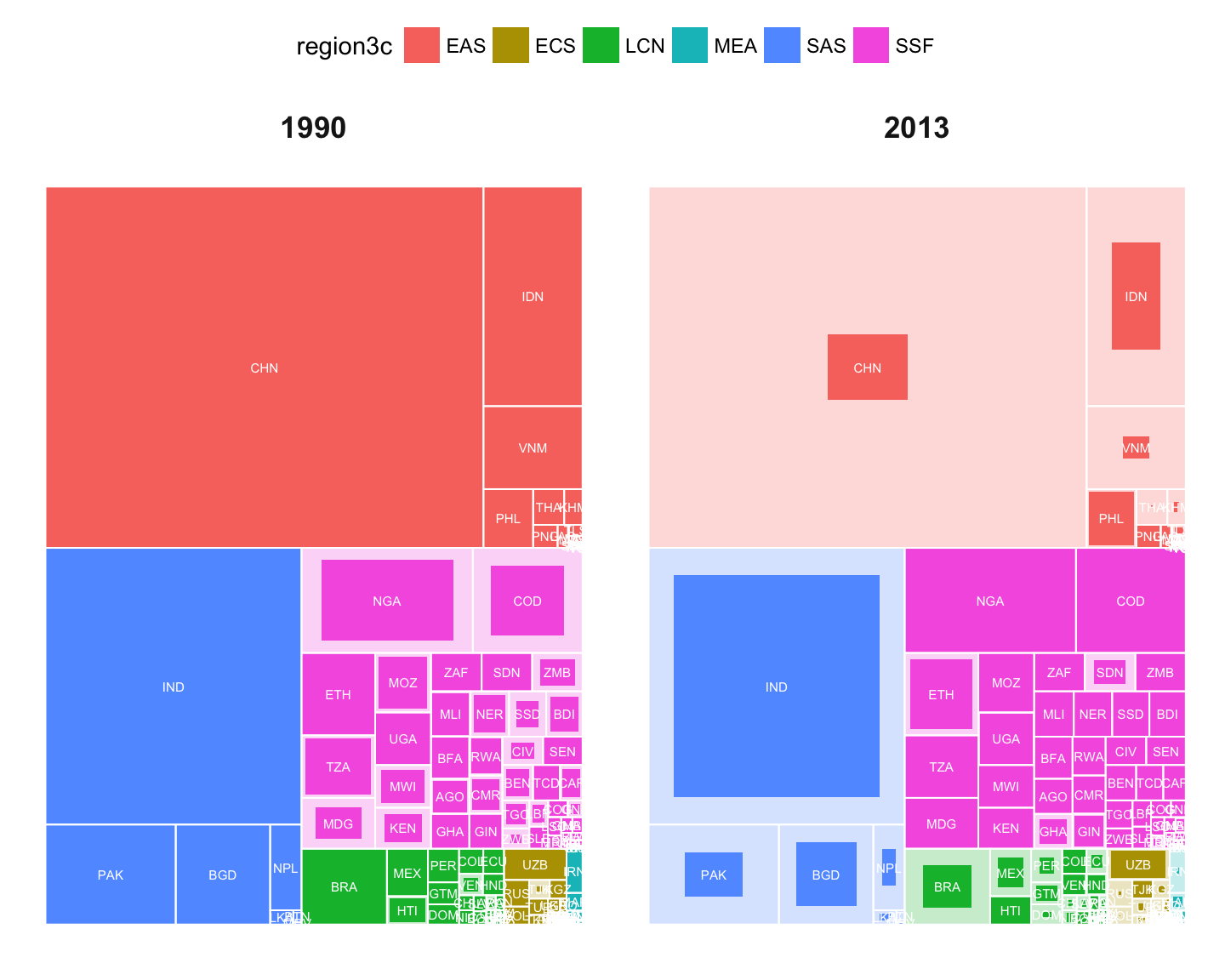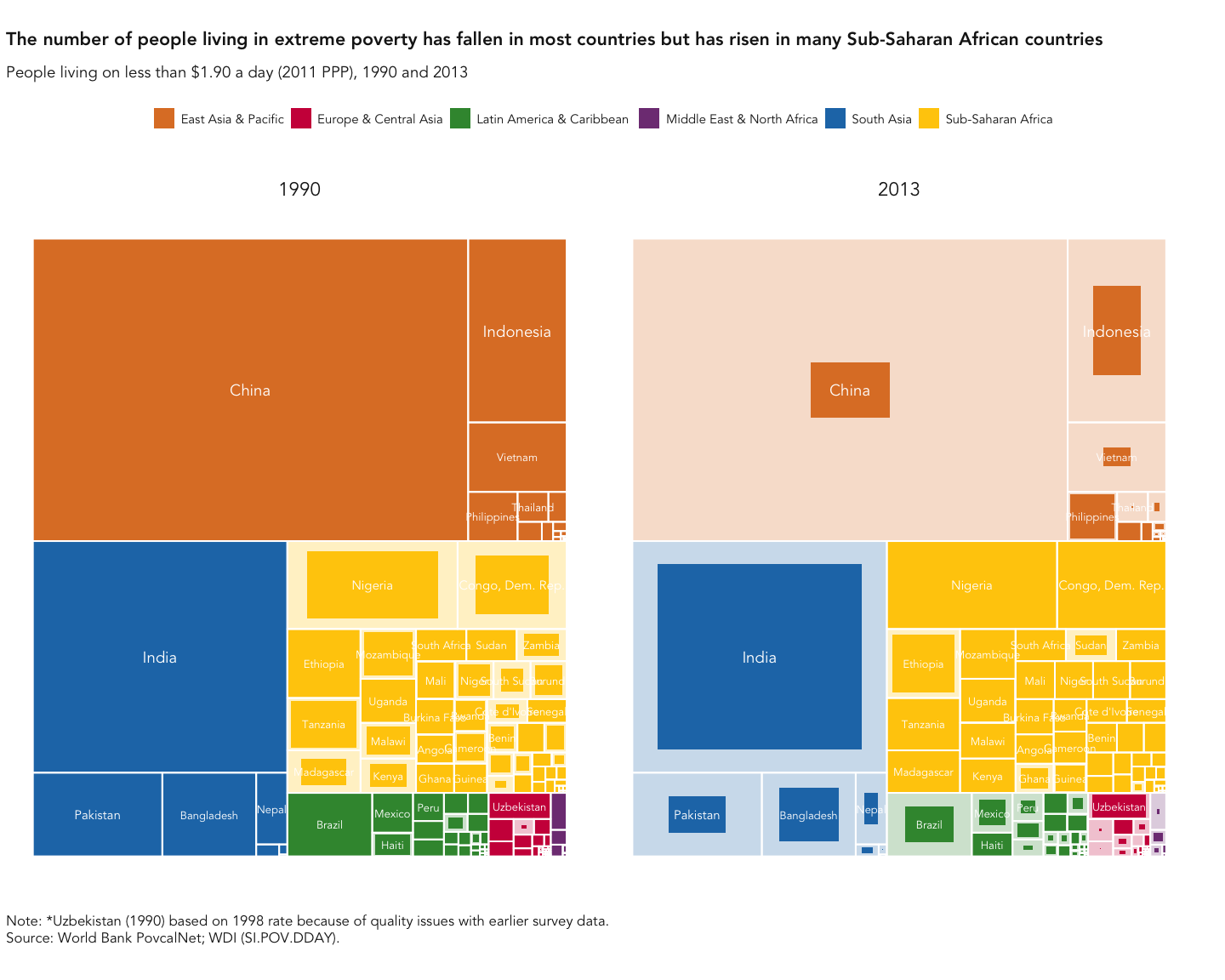# Replicating the poverty treemap

#### 2017-11-10

I made the orginal poverty treemap (pages 2 and 3 of this rather bulky PDF) by actually post-processing the SVG generated by the treemap package, which was then hand-finished by graphic designers (you can read a little about that process here).

That wasn’t an approach designed to be reuseable, whereas this one is, and should make it relatively easy to produce a reasonably good-looking comparative treemap using ggplot2.

## The side-by-side comparative form

We can use the data from the D3 animated treemap to make a first cut of the comparative treemaps. First we load the data and transform it as required.

library(tidyr)
library(dplyr)
library(ggplot2)
library(ggtreemap)

xp <- read.csv("https://worldbank.github.io/wdi-sdg/sdg1/extreme_poverty.csv", stringsAsFactors = FALSE)

xp <- xp %>%
gather("year", "num_poor", year_1990:year_2013) %>%
mutate(year = substr(year, 6, 10))

# Generate the 2 year dataset
xp_2yr <- xp %>%
filter(year %in% range(year))

# Generate max of the two years
xp_max_2yr <- xp_2yr %>%
group_by(iso3c) %>%
summarise(year_max_2yr = max(num_poor))

xp_2yr <- xp_2yr %>% left_join(xp_max_2yr)

knitr::kable(head(xp_2yr))
country iso3c region income_FY17 region3c year_max year num_poor year_max_2yr
Albania ALB Europe & Central Asia Upper middle income ECS 0.098898 1990 0.003619 0.029580
Angola AGO Sub-Saharan Africa Upper middle income SSF 7.626078 1990 5.170998 6.434680
Argentina ARG Latin America & Caribbean High Income LCN 4.745408 1990 0.250536 0.680750
Armenia ARM Europe & Central Asia Lower middle income ECS 0.943263 1990 0.384444 0.384444
Azerbaijan AZE Europe & Central Asia Upper middle income ECS 0.524576 1990 0.044392 0.044392
Bangladesh BGD South Asia Lower middle income SAS 49.732098 1990 45.083893 45.083893

Then we can make a basic version of the treemap using standard ggplot.

# Only label those with > 0.25% of the max
np.total <- sum(xp_max_2yr$year_max_2yr) iso3c_labelled <- xp_max_2yr$iso3c[xp_max_2yr$year_max_2yr / np.total > 0.0025] ar <- 0.75 base_plot <- ggplot(xp_2yr, aes(area = num_poor, layout_area = year_max_2yr, fill = region3c, subgroup = region3c)) + geom_rect(aes(area = year_max_2yr), stat = "treemap", aspect.ratio = ar, color = NA, alpha = 0.25) + geom_rect(stat = "treemap", aspect.ratio = ar, color = NA) + geom_rect(aes(area = year_max_2yr), stat = "treemap", aspect.ratio = ar, color = "white", fill=NA, size = 0.35) + scale_y_reverse() + facet_wrap(~ year) + guides(fill = guide_legend(nrow = 1, direction = "horizontal")) base_plot + geom_text(aes(label = iso3c),stat = "treemap", aspect.ratio = ar,color = "white", size = 2) + theme_minimal() + theme(axis.text = element_blank(), panel.grid = element_blank(), legend.position = "top", strip.text = element_text(size = rel(1.2), face = "bold"))And finally, we can polish it using country names and colors from wbgcharts, an internal package that is (not yet) public. It’s not perfect - labels should be dark when over light areas, and some of the longer country names should be split over multiple lines. if (require(wbgcharts)) { style <- style_atlas() finished_plot <- base_plot + geom_text( aes( label = ifelse(iso3c %in% iso3c_labelled, wbgref$countries$labels[iso3c], ""), size = cut(year_max_2yr,c(0,50,100,Inf)) ), stat = "treemap", aspect.ratio = ar, color = "white", family = style$theme()$text$family
) +
scale_size_manual(values = c(0.7,0.8,1)*style$gg_text_size, guide = "none") + scale_fill_manual(values = style$colors$regions, labels = wbgref$regions$labels) + labs( title = "The number of people living in extreme poverty has fallen in most countries but has risen in many Sub-Saharan African countries", subtitle = "People living on less than$1.90 a day (2011 PPP), 1990 and 2013",
caption = "Note: *Uzbekistan (1990) based on 1998 rate because of quality issues with earlier survey data.\nSource: World Bank PovcalNet; WDI (SI.POV.DDAY)."
) +
style\$theme() +
theme(axis.text = element_blank(), panel.grid = element_blank(),
legend.position = "top", strip.text = element_text(size = rel(1.2), face = "bold"))
print(finished_plot)
}